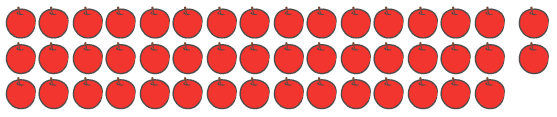### Division algorithm

The division algorithm involves repeatedly subtracting large chunks from a number, which are multiples of the number we are dividing by, and keeping track of how many multiples.

If we want to know how many 'lots of' 3 apples we can make from 47 apples we can draw a picture.We can see that there are 15 lots of 3 and 2 left over. We write 47 ÷ 3 = 15 remainder 2.

We could set this out using the division algorithm. We set the question out as follows:

 1 5 rem 2 3 ) 4 7 3 0 1 7 1 5 2

We say '3 goes into 4 once', so we write a '1' above the line.

3 × 10 = 30, so we subtract 30 from 47.

We say '3 goes into 17 five times', so we write a '5' above the line.

3 × 5 = 15, so we subtract 15 from 17.

There is a remainder of 2, so we write 'rem 2' above the line.